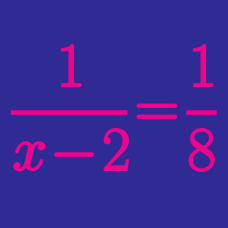Algebra

# Rational Functions - Intercepts

What is the $x$-intercept in the graph of $y=\frac{36}{x}-4?$

Let $a$ and $b$ be the $x$-intercept and $y$-intercept, respectively, in the graph of $y=\frac{2x+32}{x-4}.$ What is the value of $ab$?

What is the $y$-intercept in the graph of $y=\frac{5x+40}{x+5}?$

What is the $y$-intercept in the graph of $y=\frac{20}{x+4}+9?$

What is the $x$-intercept in the graph of $y=\frac{8}{x}-4?$

×